# common factor

H.C.F- The highest common factor of two or more given number is the highest (or greatest) of their common factor.
e.g. 24 = 1,2,3,4,6,8,12,24
36 = 1,2,3,4,6,9,12,36
H.C.F = 6

Method Factorization: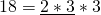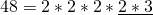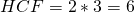Euclid’s Algorithm
determining the HCF of 18 & 30.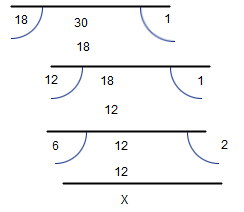Lowest /least common multiple- the lowest common multiple of two numbers is smallest natural number which is a multiple of both the number.
Example LCM of 4 an d 6
Multiple of 4 are 8 12 16 20
Multiple of 6 are 12 18 24
So LCM of 4 and 6 are 12

Method factorization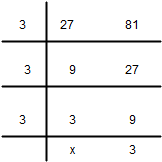So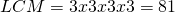The product of the H.C.F & L.C.M of two numbers is equal to the product of the number.
Example:
Given number 16 and 24.
their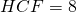andthenAbsolute value of integer |-5| = 5, but -|5| = 5, |+5| = 5